# Round table

Round table with diameter d = 105 cm is coated by square tablecloth with a side length 121 cm.

About how many cm is higher center of tablecloth than its cornes?

x =  33.1 cm

### Step-by-step explanation: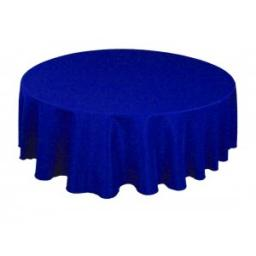Did you find an error or inaccuracy? Feel free to write us. Thank you!Tips to related online calculators
Pythagorean theorem is the base for the right triangle calculator.

#### You need to know the following knowledge to solve this word math problem:

We encourage you to watch this tutorial video on this math problem:

## Related math problems and questions:

• Round table tablecloth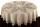The round table has an area of 50.24 dm2. Calculate the circular tablecloth diameter, if it extends beyond the edge of the table by 30 cm.
• Round table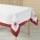A tablecloth should be sewn on a round table with a diameter of 78 cm, which should extend around the table by 10 cm. How many cms of ribbons need to be bought for edging?
• Tree trunk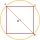What is the smallest diameter of a tree trunk that we can cut a square-section square with a side length of 20 cm?
• Tiles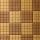How many square tiles with an area of 121 cm2 have to be ordered for the square room's paving with a side length of 2.75 meters?
• Circumscribed circle to squareFind the length of a circle circumscribing a square of side 10 cm. Compare it to the perimeter of this square.
• Again saw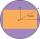From the trunk of the tree we have to a sculpture beam with rectangular cross-section with dimensions 146 mm and 128 mm. What is the trunk smallest diameter?
• DiagonalsA diagonal of a rhombus is 20 cm long. If it's one side is 26 cm, find the length of the other diagonal.
• MO circlesJuro built the ABCD square with a 12 cm side. In this square, he scattered a quarter circle with a center at point B passing through point A and a semicircle l that had a center at the center of the BC side and passed point B. He would still build a circl
• Circle and squareAn ABCD square with a side length of 100 mm is given. Calculate the radius of the circle that passes through the vertices B, C and the center of the side AD.
• ABCD squareIn the ABCD square, the X point lies on the diagonal AC. The length of the XC is three times the length of the AX segment. Point S is the center of the AB side. The length of the AB side is 1 cm. What is the length of the XS segment?
• Square diagonalCalculate the length of the square diagonal if the perimeter is 172 cm.
• Circle chordWhat is the length x of the chord circle of diameter 115 m if the distance from the center circle is 11 m?
• Circle chordCalculate the length of the chord of the circle with radius r = 10 cm, length of which is equal to the distance from the center of the circle.
• IS trapezoidCalculate the length of diagonal u and height v of isosceles trapezoid ABCD, whose bases have lengths a = |AB| = 37 cm, c = |CD| = 29 cm and legs b = d = |BC| = |AD| = 28 cm.
• Dig water wellMr. Zeman digging a well. Its diameter is 120 cm and plans to 3.5 meters deep. How long (at least) must be a ladder, after which Mr. Zeman would have eventually come out?
• Chord distanceThe circle k (S, 6 cm), calculate the chord distance from the center circle S when the length of the chord is t = 10 cm.
• Two chordsIn a circle with radius r = 26 cm two parallel chords are drawn. One chord has a length t1 = 48 cm and the second has a length t2 = 20 cm, with the center lying between them. Calculate the distance of two chords.